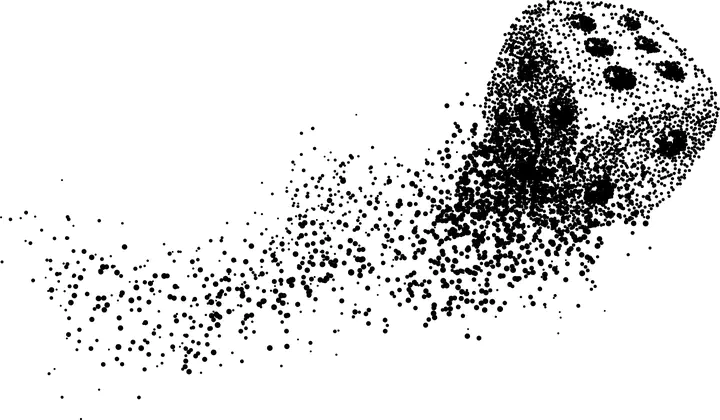# Unbounded-Time Safety Verification of Stochastic Differential DynamicsImage credit: Freepik

### Abstract

In this paper, we propose a method for bounding the probability that a stochastic differential equation (SDE) system violates a safety specification over the infinite time horizon. SDEs are mathematical models of stochastic processes that capture how states evolve continuously in time. They are widely used in numerous applications such as engineered systems (e.g., modeling how pedestrians move in an intersection), computational finance (e.g., modeling stock option prices), and ecological processes (e.g., population change over time). Previously the safety verification problem has been tackled over finite and infinite time horizons using a diverse set of approaches. The approach in this paper attempts to connect the two views by first identifying a finite time bound, beyond which the probability of a safety violation can be bounded by a negligibly small number. This is achieved by discovering an exponential barrier certificate that proves exponentially converging bounds on the probability of safety violations over time. Once the finite time interval is found, a finite-time verification approach is used to bound the probability of violation over this interval. We demonstrate our approach over a collection of interesting examples from the literature, wherein our approach can be used to find tight bounds on the violation probability of safety properties over the infinite time horizon.

Type
Publication
In CAV 2020##### Mingshuai Chen
###### ZJU100 Young Professor

My research interests include formal verification, programming theory, and logical aspects of computer science.